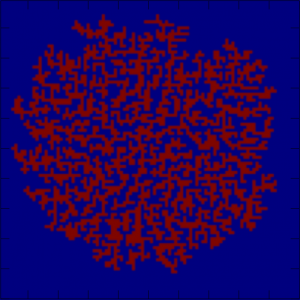# Topologically constrained models of statistical physics.

$$\def\Real{\mathbb{R}} \def\Int{\mathbb{Z}} \def\Comp{\mathbb{C}} \def\Rat{\mathbb{Q}} \def\Field{\mathbb{F}} \def\Fun{\mathbf{Fun}} \def\e{\mathbf{e}} \def\f{\mathbf{f}} \def\bv{\mathbf{v}} \def\blob{\mathcal{B}}$$

### The Blob

Consider the following planar “spin model”: the state of the system is a function from $$\Int^2$$ into $$\{0,1\}$$ (on and off states). We interpret the site $$(i,j), i,j\in\Int$$ as the plaque, i.e. the (closed) square given by the inequalities $$s_{ij}:=i-1/2\leq x\leq i+1/2; j-1/2\leq y\leq j+1/2$$.

To any configuration $$\eta$$ we associate the corresponding active domain,
$A_\eta=\bigcup_{(i,j): \eta(i,j)=1} s_{ij}.$

We are interested in the statistical ensembles supported by the finite contractible active domains – let’s refer to such domains as blobs. In other words, blobs are finite collections of plaques that are topologically equivalent to a disk. We will denote the collection of blobs as $$\blob$$.

It is worth remarking that the contractibility is a natural condition if one is interested in local models of statistical physics – such that the transition rates between the states can be defined in terms of local factors, – unlike, say, connectivity. More precisely, one can define a (symmetric) collection of transitions between states $$\eta,\eta’$$ differing at just one site $$\sigma$$ such that the feasibility of the transitions depends only on the intersections of $$\eta,\eta’$$ with a $$3\times 3$$ vicinity of $$\sigma$$, such that $$\eta’$$ is a blob iff $$\eta$$ is a blob, and turning $$\blob$$ into a connected graph (which we still refer to as $$\blob$$).

Given a fugacity $$\rho$$, on can turn $$\blob$$ into a Markov chain, by setting the jump rate adding a site as $$\rho$$, and the jump rate removing a site as $$1$$. We will denote this Markov chain as $$\blob(rho)$$.

There are several connections of the blob to the staple models of statistical physics. In essence, we are looking at the Ising model with just one contour. The standard constructions pertaining to, say, Wulff shapes, or the general framework of abstract polymer models. Alternatively, one can consider the blob as a self-avoiding loop weighted by the area it encloses.

Whichever the connection, the model seems to be new, and deserves some attention.Shape of the blob after $$10^5$$ growth steps, with fugacity $$\rho=.9$$.

#### Size, shape and agility

Consider the subcritical fugacity $$\rho<1$$. In this case, the size of the blob is a.s. finite. What is the tail distribution of its diameter (or area)? If $$\rho=1-\epsilon$$ is close to one, what is the expected size of the blob?

Ir seems that the shape of the blob is fractal like, but at a large scale resembling a round disk. Is that true?

Macroscopically, one can expect the blob to drift, converging, upon proper rescaling to Brownian motion. What are the correct scales?

#### Spanning trees

Consider the situation where some of the nodes are stuck in the active state (equivalently, their rate of switching from ON to OFF is zero). In the limit of small fugacity $$\rho\to 0$$ the resulting blob will be approximating a certain one-dimensional complex minimizing a functional – i.e. behaving like a Steiner tree.

Can we make this intuitive picture precise?

#### Tail behavior

More on the sites stuck in the active state: assume one starts with the (infinite) configuration where the whole halfplane (say, the upper halfplane) is active. Assume that the site at the origin is always ON, and all other sites perform the usual dynamics for some small fugacity. On every compact subset, the resulting blob will look like a tail starting at the origin and meandering off to infinity. What are the properties of this blob? How many sites are typically active in a box of size $$N\times N$$?